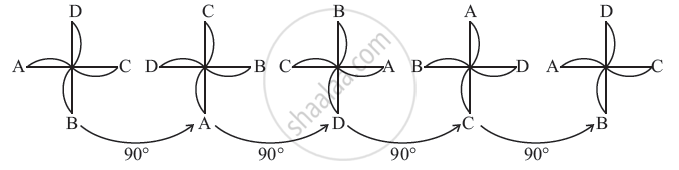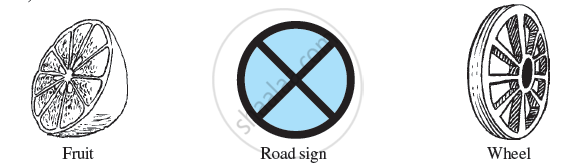Concept of Rotational Symmetry:

• Rotation turns an object about a fixed point.

• Rotation, like the movement of the hands of a clock, is called a clockwise rotation; otherwise, it is said to be anticlockwise.

• When an object rotates, its shape and size do not change.

• The rotation turns an object about a fixed point. This fixed point is the centre of rotation.

• The angle of turning during rotation is called the angle of rotation.

• A full turn, you know, means a rotation of 360°.

• A half-turn means rotation by 180°; a quarter-turn is a rotation by 90°.

• If, after a rotation, an object looks exactly the same, we say that it has rotational symmetry.

• In a complete turn (of 360 degrees), the number of times an object looks exactly the same is called the order of rotational symmetry. The order of symmetry of a square, for example, is 4 while, for an equilateral triangle, it is 3.

For Example:The Paper windmill in the picture looks symmetrical, but you do not find any line of symmetry. No folding can help you to have coincident halves. However, if you rotate it by 90° about the fixed point, the windmill will look exactly the same. We say the windmill has rotational symmetry. In a full turn, there are precisely four positions (on rotation through the angles 90°,180°, 270°, and 360°) when the windmill looks exactly the same. Because of this, we say it has rotational symmetry of order 4.

The point where we have the pin is the centre of rotation. It is the intersecting point of the diagonals in this case. Every object has rotational symmetry of order 1, as it occupies the same position after a rotation of 360° (i.e., one complete revolution). Such cases have no interest for us.

For example, when you slice certain fruits, the cross-sections are shapes with rotational symmetry.If you would like to contribute notes or other learning material, please submit them using the button below.

Shaalaa.com

What is Rotational Symmetry? [00:09:34]
S
0%